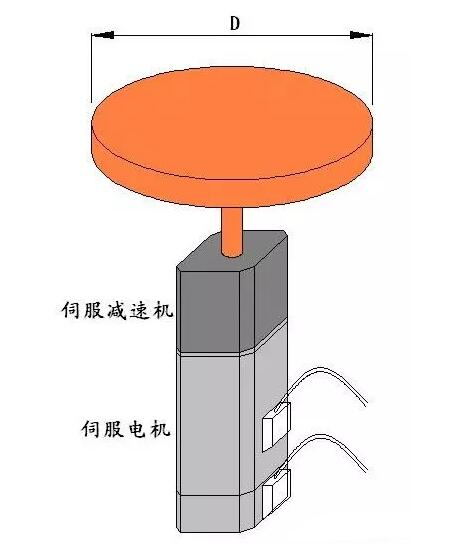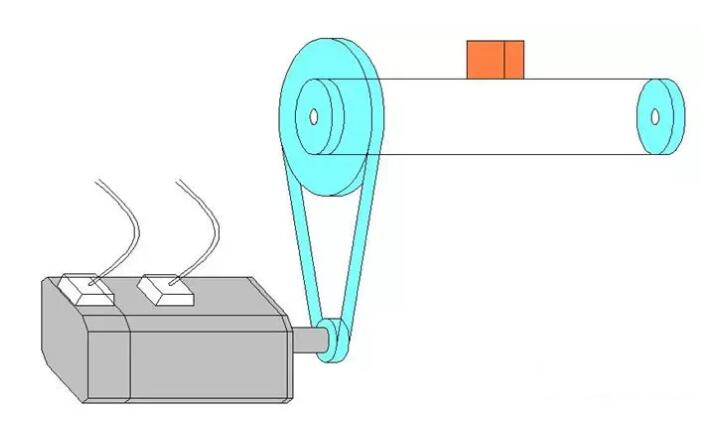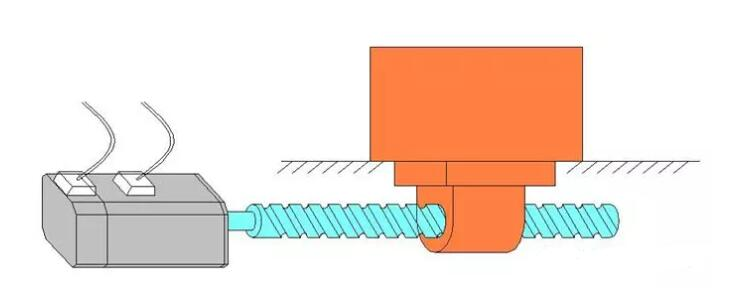伺服电机选型计算的几种常见案例JL= MD2/ 8 = 50 * 2500 / 8 = 15625kg.cm21.计算折算到电机轴上的负载惯量

JL=M * D2 / 4 / R12 =50 * 144 / 4 / 100 =18kg.cm2

JM>6kg.cm2

2.计算电机驱动负载所需要的扭矩

= 50 * 9.8 * 0.6 * 0.06 / 2 / 10

= 0.882N.m

=50 * (30 / 60 / 0.2) * 0.06 / 2 / 10

=0.375 N.m

3.计算电机所需要转速

N= v/(πD)*R1

= 30 / (3.14 * 0.12) * 10

= 796 rpm1.计算折算到电机轴上的负载惯量

=200*(2/6.28)2

=20.29kg.cm2

=40*25/8

=125kg.cm2

2.计算电机转速

=30/0.02

=1500rpm

3.计算电机驱动负载所需要的扭矩

=200*9.8*0.2*0.02/2π/0.9

=1.387N.m

=200*(30/60/0.2)*0.02/2π/0.9

=1.769N.m

=0.0125*(1500*6.28/60/0.2)/0.9

=10.903N.m

4. 选择伺服电机